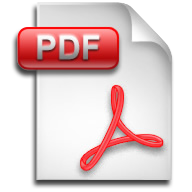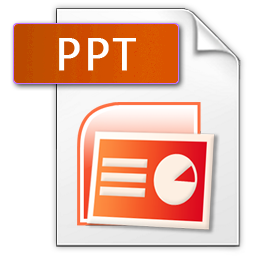# Shape and Space

The following set of questions will explore how to best compare and measure using non-standard units of measurement in relation to the volume (capacity) of objects., , , , , ,

rating(No Ratings Yet)Loading...

This set of questions explores the measurement of mass (weight) using nonstandard units of measurement, estimation and ordering., , , , , , , , , ,

rating(No Ratings Yet)Loading...

The following set of questions will explore how to best compare and measure using non-standard units of measurement in relation to the mass (weight) of objects., , , , , , ,

rating(No Ratings Yet)Loading...

The following set of questions will explore how to best compare and measure using non-standard units of measurement in relation to the length of objects., , , , , ,

rating(No Ratings Yet)Loading...

This problem set deals with the ability to understand the days of the week and months of the year., , , ,

rating(No Ratings Yet)Loading...

This problem set deals with the internal and external angles of the polygons up to an octagon., , , , , , , , ,

rating(No Ratings Yet)Loading...

Do you need to know the length of every side to calculate the area of these shapes? The following set of questions will explore strategies for finding the areas of different shapes., , , , , ,

rating(No Ratings Yet)Loading...

The following set of questions will introduce various methods for finding the perimeter of objects with missing side lengths. Ways to maximize and minimize perimeter will also be explored., , , , ,

rating(No Ratings Yet)Loading...

What is the formula to calculate the area of a triangle? This set of questions will show visually how we can find the area of triangles using only the area of rectangles., , , , , ,

rating(No Ratings Yet)Loading...

Faculty of Education
Scarfe Building
2125 Main Mall,